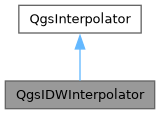Searching...
No Matches
QgsIDWInterpolator Class Reference

Inverse distance weight interpolator. More...

`#include <qgsidwinterpolator.h>`

Inheritance diagram for QgsIDWInterpolator:[legend]

## Public Member Functions

QgsIDWInterpolator (const QList< QgsInterpolator::LayerData > &layerData)
Constructor for QgsIDWInterpolator, with the specified layerData sources.

double distanceCoefficient () const
Returns the distance coefficient, the parameter that sets how the values are weighted with distance.

int interpolatePoint (double x, double y, double &result, QgsFeedback *feedback=nullptr) override
Calculates interpolation value for map coordinates x, y.

void setDistanceCoefficient (double coefficient)
Sets the distance coefficient, the parameter that sets how the values are weighted with distance.Public Member Functions inherited from QgsInterpolator
QgsInterpolator (const QList< QgsInterpolator::LayerData > &layerData)

virtual ~QgsInterpolator ()=default

QList< LayerDatalayerData () constPublic Types inherited from QgsInterpolator
enum  Result { Success = 0 , Canceled , InvalidSource , FeatureGeometryError }
Result of an interpolation operation. More...

enum  SourceType { SourcePoints , SourceStructureLines , SourceBreakLines }
Describes the type of input data. More...

enum  ValueSource { ValueAttribute , ValueZ , ValueM }
Source for interpolated values from features. More...Protected Member Functions inherited from QgsInterpolator
Result cacheBaseData (QgsFeedback *feedback=nullptr)
Caches the vertex and value data from the provider.Protected Attributes inherited from QgsInterpolator
QVector< QgsInterpolatorVertexDatamCachedBaseData
Cached vertex data for input sources.

bool mDataIsCached = false
Flag that tells if the cache already has been filled.

QList< LayerDatamLayerData
Information about the input vector layers and the attributes (or z-values) that are used for interpolation.

## Detailed Description

Inverse distance weight interpolator.

Definition at line 29 of file qgsidwinterpolator.h.

## ◆ QgsIDWInterpolator()

 QgsIDWInterpolator::QgsIDWInterpolator ( const QList< QgsInterpolator::LayerData > & layerData )

Constructor for QgsIDWInterpolator, with the specified layerData sources.

Definition at line 23 of file qgsidwinterpolator.cpp.

## ◆ distanceCoefficient()

 double QgsIDWInterpolator::distanceCoefficient ( ) const
inline

Returns the distance coefficient, the parameter that sets how the values are weighted with distance.

Smaller values mean sharper peaks at the data points. The default is a coefficient of 2.

Point values are weighted by 1 / ( distance ^ coefficient ).

setDistanceCoefficient()
Since
QGIS 3.0

Definition at line 61 of file qgsidwinterpolator.h.

## ◆ interpolatePoint()

 int QgsIDWInterpolator::interpolatePoint ( double x, double y, double & result, QgsFeedback * feedback = `nullptr` )
overridevirtual

Calculates interpolation value for map coordinates x, y.

Parameters
 x x-coordinate (in map units) y y-coordinate (in map units) result interpolation result feedback optional feedback object for progress and cancellation support
Returns
0 in case of success

Implements QgsInterpolator.

Definition at line 27 of file qgsidwinterpolator.cpp.

## ◆ setDistanceCoefficient()

 void QgsIDWInterpolator::setDistanceCoefficient ( double coefficient )
inline

Sets the distance coefficient, the parameter that sets how the values are weighted with distance.

Smaller values mean sharper peaks at the data points.

Point values are weighted by 1 / ( distance ^ coefficient ).